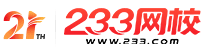一级造价工程师

• 网校课程扫描二维码进入

• 题 库扫描二维码进入

• 移动资讯233网校移动资讯

• 移动APP扫描二维码下载网校

2019一级造价工程师《造价管理》常用公式：财务评价指标

1、全部投资收益率R=(F+Y+D)/I

2、自有资金收益率Re=(F+D)/Q

3、全部投资利润率R/=(F+Y)/I

4、自有资金利润率R/=F/Q注：F：正常年销售利润(销售利润=销售收入―经营成本―折旧费―税金―利息)

Y：正常年贷款利息。I：总投资(包括基建投资和流动资金)。 D：折旧费。

5、投资收益率=(年净收益)/(项目全部投资)注：项目全部投资=固定资产投资+流动资金投资。

6、投资利润率=(税前利润总额)/(投资总额)。

7、投资利税率=(税前利润总额+销售税金及附加)/(投资总额)

8、资本金利润率=(税后利润)/(资本金)。

9、资产负债率=(负债总额)/(资产总额)

10、动比率=(流动资产总额)/(流动负债总额)

11、速动比率=(速动资产总额)/(流动负债总额)

12、借款偿还期=偿清债务年份数-1+(偿清债务当年应付的本息)/(当年可用于偿债的资金总额)

13、投资回收期=累计净现金流量现值开始出现正值的年份-1+(上一年累计现金流量现值的绝对值)/(当年净现金流量现值)

14、产权比率=(负债总额)/(股东权益)

15、有形资产净值债务率=[负债总额/(股东权益-无形资产净值)]*100%

16、 已获利息的倍数=(息前税前利润)/(利息费用)

17、销售净利率=(净利润)/(销售收入)

18、资产净利率=(净利润)/(平均资产总额)

19、净资产收益率=(净利润)/(平均所有者权益)

20、 资产负债率=(负债总额)/(资产总额)

21、利息备付率=(当期税前利润+当期利息)/(当期应付利息)。注：《计控》

22、利息备付率=(税息前利润)/(当期应付利息费用)。注：《相关》注：税息前利润=利润总额+计入总成本费用的利息费用。正常经营的企业，利息备付率应当大于2.

23、偿债备付率=(折旧+摊销+全部利息+税后利润)/(当期还本付息额)注：《计控》。

24、偿债备付率=(可用于还本付息资金)/(当期应还本付息金额)。注：《相关》。注：可用于还本付息的资金包括：可用于还款的折旧和摊销，成本中列支的利息费用，可用于还款的利润等。当期应还本付息金额包括当期应还贷款本金及计入成本的利息。应大于1.

25、 财务内部收益率(FIRR)

(1)、NPV2要用绝对值。

(2)、[i2-i1]要在3%以内，至少要在5%以内。

26、净现值率：是项目净现值与项目全部投资现值之比：NPVR=(NPV)/(Ip)

27、净年值NAV=[Σ(CI-CO)t(1+ic)-t](A/P， ic，n);或 NAV=NPV(A/P，ic，n)

2019年一级、二级造价工程师报考条件

1

2

3

移动端/互动交流
x

app下载